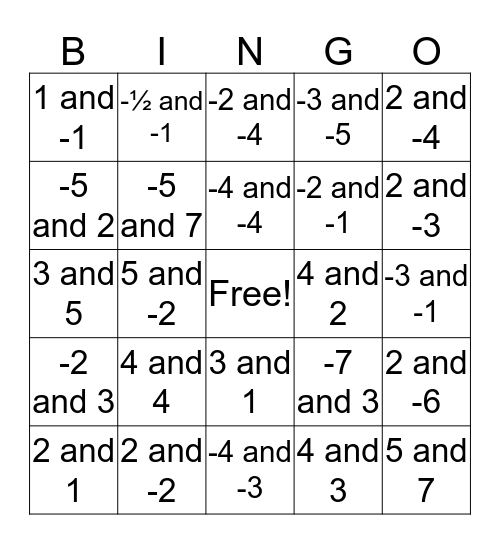# Zeros BingoThis bingo card has a free space and 24 words: 4 and 4, 2 and -2, -4 and -4, 2 and 1, 2 and -4, 1 and -1, -5 and 2, -½ and -1, 4 and 2, 3 and 1, -2 and -1, 3 and 5, -7 and 3, 2 and -6, -5 and 7, 4 and 3, -3 and -1, -2 and -4, 2 and -3, 5 and -2, 5 and 7, -2 and 3, -3 and -5 and -4 and -3.

## Play Online